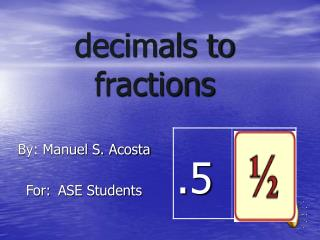DownloadDownload Presentationdecimals to fractions

# decimals to fractions

Télécharger la présentation## decimals to fractions

- - - - - - - - - - - - - - - - - - - - - - - - - - - E N D - - - - - - - - - - - - - - - - - - - - - - - - - - -
##### Presentation Transcript

1. decimals to fractions By: Manuel S. Acosta For: ASE Students

2. What is a decimal? • A decimal is a representation of a part to a whole. • .5 = 5 of 10 • The decimal point represents the one and the numbers following the decimal represent every zero that is found after the one.

3. What is a fraction? • A fraction is a representation of a part to a whole. 5 part (numerator) 10 whole (denominator) The numerator represents the part of the whole.

4. Steps to converting decimals to fractions Write the number given after the decimal. Example - 5 STEP 1

5. STEP 2 Draw a line under the number 5

6. Step 3 Add a one to replace the decimal point. 5 1

7. STEP 4 Add a zero for each number in the top line. 5 10

8. STEP 5 • Reduce the fraction created, if necessary. 5 ÷ 5 = 1 10 ÷ 5 = 2 • That is it! You have converted a decimal into a fraction!